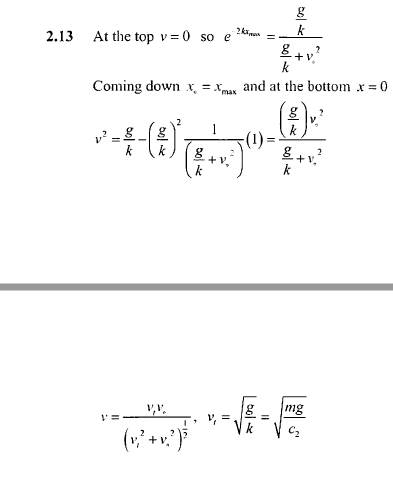# How come "terminal velocity" and "final velocity" are different?

## Homework Statement

Someone shot the bullet perpendicular to the ground. And there is air resistance.
Velocity of bullet is
v^2 = Ae^(-2kx)-g/k upward
v^2 = g/k-Be^(2kx) downward

A,B is constant, g is a gravitational acceleration, k = c2/m , c2 is a resistance constant, m is a mass of bullet.

When air resistance is C2v^2 show that final speed of the bullet is

VoVt/Root(Vo^2+Vt^2) , Vo is initial velocity and Vt is terminal velocity.

## Homework Equations

I thought that terminal velocity is the velocity when there is no net force on the object.
Since there is no parasutre, shouldn't the final velocity is same with terminal velocity?
How come final velocity is smaller than terminal velocity...
( I am pretty sure in this question we don't have to consider air density )

## The Attempt at a SolutionI did solve this mathmetically but I just don't understand the physical concept.
How can something be slower when net force on it is zero.

[/B]

#### Attachments

•